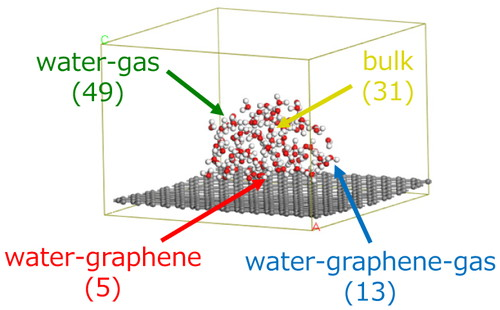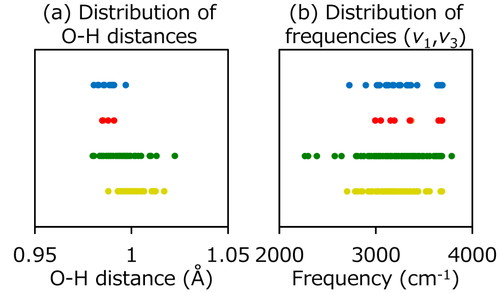Journal of Computer Chemistry, Japan
Online ISSN : 1347-3824
Print ISSN : 1347-1767
ISSN-L : 1347-1767
Letters (Selected Paper)
Theoretical Study of Hydrogen Bonds in Water Nanodroplet on Graphene
Takayoshi ISHIMOTOMichihisa KOYAMA
Author information
JOURNAL FREE ACCESS FULL-TEXT HTML

2016 Volume 15 Issue 3 Pages 85-86

Details
Abstract

We analyzed the structural differences of H2O molecules in water nanodroplet on graphene ((H2O)98 on graphene) model by using the density functional theory. 98 H2O molecules on graphene were classified into four groups based on the surrounding condition (bulk region, water-gas interface, water-graphene interface, and water-graphene-gas interface). The O–H distances and vibrational frequencies of H2O molecules near the gas region were wider distributions compared with the H2O molecules in the bulk region, whereas narrower distributions were obtained near the graphene interface.

1 Introduction

The wettability control at interface is an important issue to improve the thermal energy transfer. Recently, it was experimentally observed that the wettability on graphene surface changes with the change in the number of graphene layers . This result indicates that the electronic structure of graphene changes the wettability. In addition, the physical properties of water nanodroplet, such as boiling and melting points, drastically change in comparison with the bulk water when the size of water nanodroplet becomes small . This result indicates that the inhomogeneous hydrogen bonds in water nanodroplet affect the physical properties of water. It is expected that the understanding based on the electronic structure theory may contribute to control the wettability of interface from the atomic scale. In this study, we analyzed the geometry and vibrational frequencies of H2O in water nanodroplet on graphene by using density functional theory (DFT) to understand the properties of water near interface.

2 Theory

All DFT calculations were performed using the Vienna ab initio simulation package (VASP) [3,4] with the projector augmented wave method. The Perdew-Burke-Ernzerhof in generalized gradient approximations was used for the exchange and correlation functional. The cutoff energy was set to be 400 eV. 1 × 1 × 1 k-point was sampled by the Monkhorst-Pack grid method.

3 Results and Discussion

We first validated the accuracy of computational setting in this study. The geometrical parameters (O–H distance (R(OH)) and angle (θ(HOH))) and vibrational frequencies (symmetric stretch (v1), bending (v2), and asymmetric stretch (v3)) of isolated H2O molecule are shown Table 1.

Table 1.  Calculated geometrical parameters and vibrational frequencies of H2O molecule.
 R(OH) (Å) θ(HOH) (°) v1 (cm−1) v2 (cm−1) v3 (cm−1) This work 0.973 104.3 3733.1 1594.5 3832.7 Expt [5,6]. 0.958 104.8 3657.1 1594.7 3755.9

We clearly found that both geometrical parameters and vibrational frequencies of H2O reasonably reproduced the experimental values [5,6] by our calculation setting.

We next optimized the model structure of water nanodroplet consisting of 98 H2O molecules (ca. 2.2 nm) on graphene as shown in Figure 1. The initial structure of water nanodroplet was prepared by the Packmol . H2O molecules in nanodroplet were classified into four groups based on the surrounding condition. 31, 49, 5, and 13 H2O molecules are included in the bulk, water-gas interface, water-graphene interface, and water-graphene-gas interface, respectively.Figure 1.

Optimized structure of water-graphene interface model and classified H2O molecules. Number of H2O molecules is shown in parenthesis.

We analyzed the distributions of O–H distances and vibrational frequencies for each group of H2O. In Figure 2 (a), H2O molecules in water-gas interface showed wider distribution compared with the H2O in the bulk region. On the other hand, H2O molecules near graphene showed shorter O–H distances and narrower distributions. As well as the O–H distances, wide distribution of vibrational frequencies of H2O molecules in water-gas interface were obtained as shown in Figure 2 (b). We clearly found the relation between O–H distances and vibrational frequencies of H2O because the distributions of these values show a similar trend in the same groups. These results indicate that the nature of H2O molecules on graphene is different under the surrounding conditions, such as water-gas or water-graphene interfaces. In addition, it is expected that the H2O molecules near interfaces affect thermal transfer because the vibrational frequencies of H2O near interface are different from the bulk region.Figure 2.

Distribution of (a) O–H distances and (b) vibrational frequencies (v1 and v3) of H2O in optimized water-graphene interface model. Yellow, green, red, and blue correspond to the water in bulk region, water-gas interface, water-graphene interface, and water-graphene-gas interface, respectively.

4 Conclusions

To understand the wettability of water on graphene, we analyzed the structural difference of H2O molecules in water nanodroplet on graphene by using DFT. H2O molecules in the model were divided into four groups. The different distributions about O–H distances and vibrational frequencies were obtained in different interfaces due to the inhomogeneity of water molecules at interface.

Acknowledgment

The activities of INAMORI Frontier Research Center are supported by Kyocera Corporation. We also acknowledge the financial support from JSPS KAKENHI Grant Number 25709012. All calculations are performed on the CX400 computer systems in Research Institute for Information Technology, Kyushu University.

© 2016 Society of Computer Chemistry, Japan
Top